How to Calculate and Solve for Pressure and Initial Compressibility Factor Ratio of a Reservoir Fluid Flow | The Calculator Encyclopedia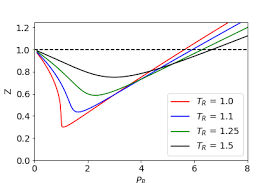The image above represents pressure and initial compressibility factor ratio (P/Z Ratio).

To compute for the pressure and initial compressibility factor ratio, four essential parameters are needed and these parameters are initial pressure (Pi), Initial Compressibility Factor (Zi), Cumulative Gas Production (GP) and initial gas in place (G).

The formula for calculating the P/Z Ratio:

P/Z = Pi / Zi (1 – Gp / G)

Where;

P/Z = P/Z Ratio
Pi = Initial Pressure
Zi = Initial Compressibility Factor
GP = Cumulative Gas Production
G = Initial Gas in Place

Let’s solve an example;
Given that the initial pressure is 20, the initial compressibility factor is 28, the cumulative gas production is 30 and the initial gas in place is 34.
Find the pressure and initial compressibility factor ratio (P/Z Ratio)?

This implies that;

Pi = Initial Pressure = 20
Zi = Initial Compressibility Factor = 28
GP = Cumulative Gas Production  = 30
G = Initial Gas in Place = 34

P/Z = Pi / Zi (1 – Gp / G)
P/Z = 20 / 28 (1 – 30 / 34)
P/Z = 20 / 28 (1 – 0.88)
P/Z = 20 / 28 (0.117)
P/Z = 0.714(0.117)
P/Z = 0.084

Therefore, the P/Z Ratio is 0.084.

Calculating for Initial Pressure when P/Z Ratio, Initial Compressibility Factor, Cumulative Gas Pressure and Initial Gas In Place is Given.

Pi = P/Z x Zi / (1 – Gp / G)

Where;

Pi = Initial Pressure
P/Z = P/Z Ratio
Zi = Initial Compressibility Factor
GP = Cumulative Gas Production
G = Initial Gas in Place

Let’s solve an example;
Given that the P/Z Ratio is 50, the initial compressibility factor is 18, the cumulative gas production is 25 and the initial gas in place is 32.
Find the initial pressure ?

This implies that;

P/Z = P/Z Ratio = 50
Zi = Initial Compressibility Factor = 18
GP = Cumulative Gas Production = 25
G = Initial Gas in Place = 32

Pi = P/Z x Zi / (1 – Gp / G)
Pi = 50 x 18 / (1 – 25 / 32)
Pi = 900 / (1 – 0.78125)
Pi = 900 / (0.21875)
Pi = 4114.28

Therefore, the Initial Pressure is 4114.28.

Calculating for Initial Compressibility Factor when P/Z Ratio, Initial Pressure, Cumulative Gas Pressure and Initial Gas In Place is Given.

Zi = Pi (1 – Gp / G) / P/Z

Where;

Zi = Initial Compressibility Factor
Pi = Initial Pressure
P/Z = P/Z Ratio
GP = Cumulative Gas Production
G = Initial Gas in Place

Let’s solve an example;
Given that the P/Z Ratio is 40, the initial pressure is 18, the cumulative gas production is 15 and the initial gas in place is 22.
Find the initial compressibility factor ?

This implies that;

Pi = Initial Pressure = 18
P/Z = P/Z Ratio = 40
GP = Cumulative Gas Production = 15
G = Initial Gas in Place = 22

Zi = Pi (1 – Gp / G) / P/Z
Zi = 18 (1 – 15 / 22) / 40
Zi = 18 (1 – 0.681) / 40
Zi = 18 (0.319) / 40
Zi = 5.742 / 40
Zi = 0.1435

Therefore, the initial compressibility factor is  0.1435.

How to Calculate and Solve for Gas Initially in Place of a Reservoir Fluid Flow | The Calculator Encyclopedia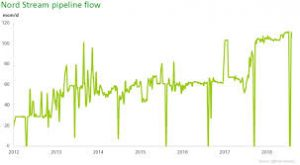The image above represents gas initially in place.

To compute for gas initially in place, five essential parameters are needed and these parameters are drainage area (A), initial gas FVF (Bgi), initial water saturation (Swi), porosity (φ) and thickness (h).

The formula for calculating gas initially in place:

G = 43560Ah φ (1 – Swi) / Bgi

Where;

G = Gas Initially in Place
A = Drainage Area
Bgi = Initial Gas FVF
Swi = Initial Water Saturation
φ = Porosity
h = Thickness

Let’s solve an example;
Find the gas initially in place with a drainage area of 44, initial gas FVF of 23, initial water saturation of 32, porosity of 18 and exponent of 14.

This implies that;

A = Drainage Area = 44
Bgi = Initial Gas FVF = 23
Swi = Initial Water Saturation = 32
φ = Porosity = 18
h = Thickness = 14

G = 43560Ah φ (1 – Swi) / Bgi
G = 43560 x 44 x 14 x 18 (1 – 32) / 23
G = 43560 x 44 x 14 x 18 (-31) / 23
G = -14972791680 / 23
G = -650990942.6

Therefore, the gas initially in place is -650990942.6

How to Calculate and Solve for Gas Flow of Reservoir Fluid Flow | The Calculator Encyclopedia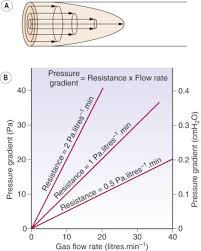The image above represents gas flow.

To compute for the gas flow, four essential parameters are needed and these parameters are Performance Co-efficient (C), Average Reservoir Pressure (Pr*), Flowing Bore Hole Pressure (Pwf*) and Exponent (n).

The formula for calculating the gas flow:

Qg = C(Pr*² – Pwf*²)n

Where;

Qg = Gas Flow
C = Performance Co-efficient
Pr* = Average Reservoir Pressure
Pwf* = Flowing Bore Hole Pressure
n = Exponent

Let’s solve an example;
Find the gas flow when a performance Co-efficient is 9, average reservoir pressure is 10, flowing bore hole pressure is 5 and an exponent of 20 is given.

This implies that;

C = Performance Co-efficient = 9
Pr* = Average Reservoir Pressure = 10
Pwf* = Flowing Bore Hole Pressure = 5
n = Exponent = 20

Qg = C(Pr*² – Pwf*²)n
Qg = 9(10² – 5²)20
Qg = 9(100 – 25)20
Qg = 9(75)20
Qg = 9(3.17e+37)
Qg = 2.85e+38

Therefore, the gas flow is 2.85e+38.

How to Calculate and Solve for Total Compressibility of a Reservoir Fluid Flow | The Calculator Encyclopedia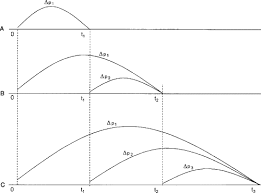The image above represents the total compressibility.

To compute for the total compressibility, seven essential parameters are needed and these parameters are Water compressibility (Cw), Oil compressibility (Co), Gas compressibility (Cg), Rock compressibility (Cr), water saturation (Sw), Oil saturation (So) and Gas saturation (Sg).

The formula for calculating the total compressibility:

CT = CoSo + CgSg + CwSw + Cr

Where;

CT = Total Compressibility
Cw = Water Compressibility
Co = Oil Compressibility
Cg = Gas Compressibility
Cr = Rock Compressibility
Sw = Water Saturation
So = Oil Saturation
Sg = Gas Saturation

Let’s solve an example;
With a given value of 13 as water compressibility, 17 as oil compressibility, 19 as gas compressibility, 25 as rock compressibility, 24 as water saturation, 29 as oil saturation and 21 as gas saturation.
Find the total compressibility?

This implies that;

Cw = Water Compressibility = 13
Co = Oil Compressibility = 17
Cg = Gas Compressibility = 19
Cr = Rock Compressibility = 25
Sw = Water Saturation = 24
So = Oil Saturation = 29
Sg = Gas Saturation = 21

CT = CoSo + CgSg + CwSw + Cr
CT = (17 x 29) + (19 x 21) + (13 x 24) + 25
CT = 493 + 399 + 312 + 25
CT = 1229

Therefore, the total compressibility is 1229 psi.

How to Calculate and Solve for Reservoir Radius and Well Spacing | The Calculator Encyclopedia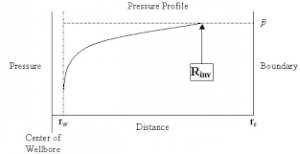The image above represents the reservoir radius.

To compute for the reservoir radius, one essential parameter is needed and this parameter is Well Spacing (A).

The formula for calculating the reservoir radius:

re = √[43560A  / π]

Where;

re = Reservoir Radius
A = Well Spacing

Let’s solve an example;
Given that the well spacing of an reservoir radius is 42. Find the reservoir radius?

This implies that;

A = Well Spacing = 42

re = √[43560A  / π]
re = √[43560 x 42 / π]
re = √[1829520 / π]
re = √582354.3
re = 763.12

Therefore, the reservoir radius is 763.12 ft.

How to Calculate and Solve for Linear Flow Rate in Reservoir Fluid Flow | The Calculator Encyclopedia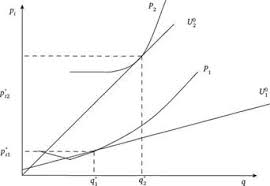The image above represents the linear flow rate.

To compute for the linear flow rate, four parameters are needed and these parameters are Initial pressure (P1), Final Pressure (P2), Thickness (h) and Permeability (k).

The formula for calculating linear flow rate:

q = 0.001127kh[P1 – P2]

Where:
q = Linear Flow Rate
P1 = Initial Pressure
P2 = Final Pressure
h = Thickness
k = Permeability

Let’s solve an example;
Find the linear flow rate when the initial pressure is 12, final pressure is 22, thickness is 18 and permeability is 44.

This implies that;
P1 = Initial Pressure = 12
P2 = Final Pressure = 22
h = Thickness = 18
k = Permeability = 44

q = 0.001127kh [P1 – P2]
q = 0.001127 x 44 x 18 [12 – 22]
q = 0.001127 x 44 x 18 [-10]
q = 0.892584 [-10]
q = -8.92584

Therefore, the linear flow rate is -8.92584 bbl/day.

Calculating Permeability when the linear flow rate, Initial Pressure, Final Pressure and Thickness is Given.

k = q / 0.001127h (p1 – p2)

Where;
k = Permeability
q = Linear Flow Rate
P1 = Initial Pressure
P2 = Final Pressure
h = Thickness

Let’s solve an example;
Find the permeability when the initial pressure is 34, final pressure is 24, thickness is 12 and linear flow rate is 50.

This implies that;
q = Linear Flow Rate = 50
P1 = Initial Pressure = 34
P2 = Final Pressure = 24
h = Thickness = 12

k = q / 0.001127h (p1 – p2)
k = 50 / 0.001127h (34 – 24)
k = 50 / 0.001127h (10)
k = 50 / 0.01127
k = 4436.5

Therefore, the permeability is 4436.5.

How to Calculate and Solve for Fluid Potential, Pressure, Datum Level and Density | The Calculator Encyclopedia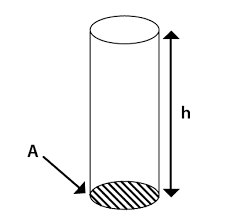The image above represents the fluid potential.

To compute for the fluid potential, three essential parameters are needed and these parameters are pressure (P), Datum Levels (ΔZ) and Density (ρ).

The formula for calculating fluid potential:

φ = P – [ρ / 144]ΔZ

Where;
φ = Fluid Potential
P = Pressure
ΔZ = Datum Levels
ρ = Density

Let’s solve an example;
Find the fluid potential when the pressure is 24 with a datum level of 18 and the density of 30.

This implies that;
P = Pressure = 24
ΔZ = Datum Levels = 18
ρ = Density = 30

φ = P – [ρ / 144]ΔZ
φ = 24 – [30/144] 18
φ = 24 – [0.2083] 18
φ = 24 – 3.75
φ = 20.25

Therefore, the fluid potential is 20.25.

Calculating the Pressure(P) when the fluid potential, Datum levels and Density is Given.

P = φ – [ρ / 144]ΔZ

Where;
P = Pressure
φ = Fluid Potential
ΔZ = Datum Levels
ρ = Density

Lets solve an example;
Find the pressure with a fluid potential of 40 and a datum levels of 18 with density of 24.

This implies that;
φ = Fluid Potential = 40
ΔZ = Datum Levels = 18
ρ = Density = 24

P = φ – [ρ / 144]ΔZ
P = 40 – [24 / 144]18
P = 40 – [0.167]18
P = 40 – 3
P = 37

Therefore, the pressure is 37.

How to Calculate and Solve for True Stress | The Calculator Encyclopedia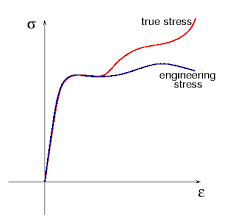The image above represents the true stress.

To compute for the true stress, two essential parameters are needed and these parameters are force (F) and instantaneous area (Ai).

The formula for calculating true stress:

σT = F / Ai

Where;
T = True Stress
F = Force
Ai = Instantaneous Area

Let’s solve an example;
Find the true stress when the instantaneous area is 60 with a force of 25.

This implies that;
F = Force = 25
Ai = Instantaneous Area = 60

σT = F / Ai
σT = 25 / 60
σT = 0.416

Therefore, the true stress is 0.416 Pa.

Calculating the Force when True Stress and Instantaneous Area is Given.

F = Ai x σT

Where;
F = Force
σT = True Stress
Ai = Instantaneous Area

Let’s solve an example;
Find the force when the instantaneous area is 30 with a true stress of 15.

This implies that;
σT = True Stress = 15
Ai = Instantaneous Area = 30

F = Ai x σT
F = 30 x 15
F = 450

Therefore, the force is 450.

How to Calculate and Solve for Road Bank Angle, Velocity and Radius of a Body in Motion of Circular Path | The Calculator Encyclopedia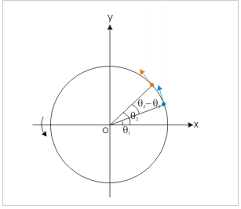The image represents road bank angle in circular motion.

To compute for the road bank angle, three essential parameters are needed and these parameters are velocity (v), acceleration due to gravity (g) and radius (r).

The formula for calculating the road bank angle;

θ = tan-1( / gr)

Where;
θ = Road Bank Angle
v = Velocity
g = Acceleration due to Gravity

Let’s solve an example;
Find the road bank angle where the acceleration due to gravity is 9.8, velocity is 35 and radius is 18.

This implies that;
v = Velocity = 35
g = Acceleration due to Gravity = 9.8
r = Radius = 18

θ = tan-1( / gr)
θ = tan-1(35² / (9.8)(18))
θ = tan-1(1225 / 176.4)
θ = tan-1(6.94)
θ = 81.81°

Therefore, the road bank angle is 81.81°.

Calculating the Velocity when Road Bank Angle, Acceleration due to Gravity and Radius is Given.

v = √gr.tan θ

Where;
v = Velocity
θ = Road Bank Angle
g = Acceleration due to Gravity

Let’s solve an example;
Given that the road bank angle is 50, radius is 15 and acceleration due to gravity is 9.8. Find the velocity?

This implies that;
θ = Road Bank Angle = 50
g = Acceleration due to Gravity = 9.8
r = Radius = 15

v = √gr.tan θ
v = √(9.8 x 15)(tan 50)
v = √(147)(1.1917)
v = √175.1799
v = 13.235

Therefore, the velocity is 13.235.

How to Calculate and Solve for Centripetal Acceleration, Angular Velocity and Radius of Circular Path of a Body | The Calculator Encyclopedia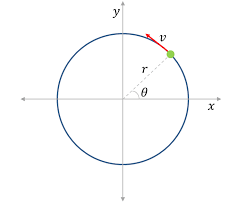The image above represents centripetal acceleration in a motion of circular path.

To compute for the centripetal acceleration, two essential parameters are needed and these parameters are angular velocity (ω) and radius of circular path (r).

The formula for the calculating centripetal acceleration:

a = ω²r

Where:
a = Centripetal Acceleration
ω = Angular Velocity
r = Radius of Circular Path

Let’s solve an example;
Find the centripetal acceleration with an angular velocity of 33 and a radius of 21.

This implies that;
ω = Angular Velocity = 33
r = Radius of Circular Path = 21

a = ω²r
a = 33² x 21
a = 1089 x 21
a = 22869

Therefore, the centripetal acceleration is 22869 m/s².

Calculating the Angular Velocity when Centripetal Acceleration and Radius of Circular path is Given.

ω = √a / r

Where:
ω = Angular Velocity
a = Centripetal Acceleration
r = Radius of Circular Path

Let’s solve an example;
Find the angular velocity with a centripetal acceleration of 320 and a radius of 8.

This implies that;
a = Centripetal Acceleration = 320
r = Radius of Circular Path = 8

ω = √a / r
ω = √320 / 8
ω = √40
ω = 6.32

Therefore, the angular velocity is 6.32.